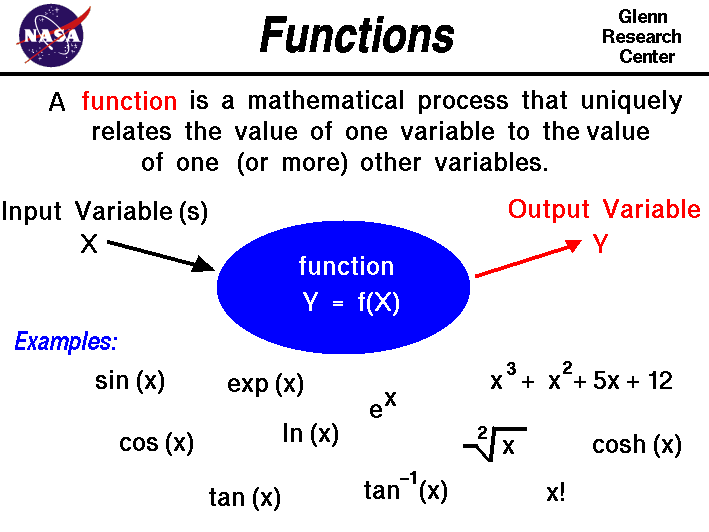+ Text Only Site
+ Non-Flash Version
+ Contact GlennAn important mathematical concept is the idea of function. A function is a mathematical process that uniquely relates the value of one variable to the value of another variable in the problem. Schematically, we can think of a function as a "processor" that takes in one (or more) input variables and produces an output variable. We call the output variable the dependent variable and the input variable is called the independent variable. Changing the value of an independent variable produces a change in the value of the dependent variable that is always the same. On this slide we will denote an independent variable as X and the dependent variable as Y. We denote that Y is a function of X by the symbol: Y = f (X) Some functions occur so often in math and science that we assign special names and symbols to them. At the bottom of the slide we have listed some examples of functions that occur in aerospace engineering. The trigonometric functions sine, cosine, and tangent relate the various sides and angles of a triangle. The value of the function depends only on the angle between two sides of a right triangle. So we can write the functions as: Y = sin(X) W = cos(X) Z = tan(X) where the value of X is an angle and the value of Y, W, and Z are numbers. Since the value of the function is always the same, the value can be tabulated and used to solve problems. Some examples of problems involving triangles and angles include the forces on a model rocket during powered flight, the application of torques, and the resolution of the components of a vector. Another function which you may have seen is the factorial function with the symbol X!. The value of this function is formed by multiplying X times X-1 times X-2, etc. until you get to 1: Y = X! Y = 4! = 4 * 3 * 2 * 1 = 24 This function occurs in many probability and statistics problems. There are some interesting properties of functions. Functions can be grouped together to form other functions. On the slide the polynomial: Y = X^3 + X^2 + 5*X + 12 is made by adding powers of X. The function here is: Y = X^n where n is any number. Y is generated by multiplying X times itself n times. There are some special functions, called inverse functions, which "un-do" the operation of some other function. The square root function is the inverse of the square function. So if Y = X^2 16 = 4^2 Then: Y = sqrt(X) 4 = sqrt(16) Since lift and drag depend on the square of the velocity, we often use square roots to solve velocity problems. This function is used in the determination of the terminal velocity of a falling object. The function tan^-1(X) on the figure is called the arc-tangent of X. This function is the inverse of the trigonometric tangent function. It returns the angle Y whose tangent is X. There are inverses for the sine and cosine as well. The exponential function, exp(X) or e^X, is a special function that comes from calculus. In calculus, we are often trying to determine the rate at which some function changes. The rate is expressed as another function called a differential and the rate is the slope of the graph of the function. If we have a function Y = f(X), then the slope of the function is called Z and; Z = dY/dX The exp function is the special function whose slope (rate of change) is equal to the value of the function. Y = exp(X) = d[exp(X)]/dX = dY/dX This function often occurs in nature when the rate of change of a variable equals the amount of the variable. The change in atmospheric pressure with altitude is an exponential. The inverse of the exponential function is the logarithmic function with the symbol ln(X). The ln function appears in many thermodynamics problems, such as calculating the change in the entropy of a gas during a thermodynamic process. The cosh (X) function on the slide is the hyperbolic cosine function. It is a special, tabulated function that results from solving a certain form of differential equation and is similar to the trigonometric cos function. This function appears in the solution to the problem of a line that sags under its own weight like the support cables of a bridge. Activities: Guided Tours Navigation ..Beginner's Guide Home Page+ Inspector General Hotline + Equal Employment Opportunity Data Posted Pursuant to the No Fear Act + Budgets, Strategic Plans and Accountability Reports + Freedom of Information Act + The President's Management Agenda + NASA Privacy Statement, Disclaimer, and Accessibility CertificationEditor: Nancy Hall NASA Official: Nancy Hall Last Updated: May 05 2015 + Contact Glenn